Vectors and conics

This free course is available to start right now. Review the full course description and key learning outcomes and create an account and enrol if you want a free statement of participation.

Free course

3.3 Equation of a plane in three-dimensional Euclidean space

We stated in Section 1.7 that the general form of the equation of a plane in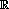3 is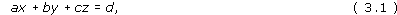where a, b and c are not all zero. We can prove this now, using the dot product.

How can we specify a plane uniquely in3? One possibility is to specify three points that lie in the plane. However, this does not enable us to prove equation (3.1) without a significant amount of algebra; so we adopt a different approach.

For a given plane in3, there is a particular direction that can be used to specify the plane – the direction perpendicular to all the vectors in that plane.

Definition

A vector that is perpendicular to all the vectors in a given plane is called a normal vector to the plane.

Note: A normal vector to a plane is also called a normal to the plane, and its direction is said to be normal to the plane.

If n is a normal vector to a given plane, then so is kn, for any non-zero real number k. If k > 0, then kn is in the same direction as n, whereas if k < 0, then kn is in the opposite direction to n.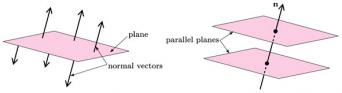A normal vector n does not determine a plane uniquely, as there are infinitely many planes that have n as a normal; these planes are parallel to one another. However, if we specify both a normal vector and a point that lies in the plane, then the plane is determined uniquely.

Example 6

Determine the equation of the plane in3 that contains the point P(2, 3, 4) and has n = (1, 2, −1) as a normal.

If the point Q(x, y, z) lies in the plane, then the vector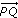must be perpendicular to the normal vector n; it follows that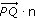must be zero.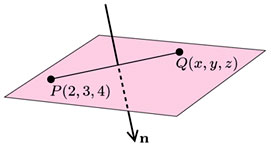Hence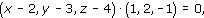so

(x − 2) × 1 + (y − 3) × 2 + (z − 4) × (−1) = 0.

This equation can be rearranged in the form

x + 2y − z = 4;

this is the equation of the plane.

In general, let n = (a, b, c) be normal to a given plane that contains the point P(x1, y1, z1). Then an arbitrary point Q(x, y, z) lies in the plane if and only if the vectorsand n are orthogonal; that is, if and only if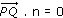.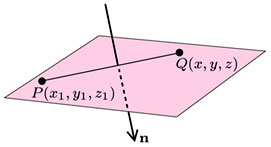Since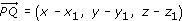, this condition can be written in the form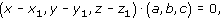so

(x − x1) × a + (y − y1) × b + (z − z1) × c = 0.

This equation can be rearranged in the form

ax + by + cz = ax1 + by1 + cz1,

that is,

ax + by + cz = d,

where d = ax1 + by1 + cz1.

Theorem 1

The equation of the plane that contains the point (x1, y1, z1)

and has n = (a, b, c) as a normal is

ax + by + cz = d,

where d = ax1 + by1 + cz1.

Once we know the equation of a plane, we can ‘read off’ the components of a normal vector as they are the coefficients of x, y and z in the equation. For instance, one normal to the plane with equation x − 2y + 3z = 7 is n = (1, −2, 3).

We shall not make use of the formula for d given in the above theorem. In practice, it is simpler to use the following corollary.

Corollary to Theorem 1

The equation of the plane that contains the point (x1, y1, z1)

and has n = (a, b, c) as a normal is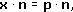where x = (x, y, z) and p = (x1, y1, z1).

Proof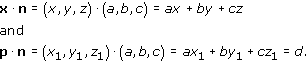Since ax + by + cz = d, from Theorem 1, it follows that x · n = p · n.

Example 7

Determine the equation of the plane in3 that contains the point (1, −1, 4) and has (2, −2, 3) as a normal.

The equation of the plane iswhere x = (x, y, z), p = (1, −1, 4) and n = (2, −2, 3); in other words, the equation of the plane is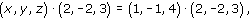that is,

2x − 2y + 3z = 1 × 2 + (−1) × (−2) + 4 × 3,

or

2x − 2y + 3z = 16.

Example 45

Determine the equation of each of the following planes:

• (a) the plane that contains the point (1, 0, 2) and has (2, 3, 1) as a normal;

• (b) the plane that contains the point (−1, 1, 5) and has (4, −2, 1) as a normal.

We use the formula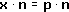for the equation of a plane, where x = (x, y, z), n is a normal to the plane and p is a point in the plane.

• (a) Here n = (2, 3, 1) and p = (1, 0, 2), so the equation of the plane is

•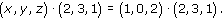• This can be expressed in the form

• 2x + 3y + z = 1 × 2 + 0 × 3 + 2 × 1,

• that is,

• 2x + 3y + z = 4.

• (b) Here n = (4, −2, 1) and p = (−1, 1, 5), so the equation of the plane is

•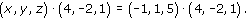• This can be expressed in the form

• 4x − 2y + z = (−1) × 4 + 1 × (−2) + 5 × 1,

• that is,

• 4x − 2y + z = −1.

We can use the general form of the equation of a plane to find the equation of a plane that contains three given points, as follows. We assume that the equation of the plane is ax + by + cz = d, and then substitute the coordinates of the three points in turn into this equation; this gives three simultaneous equations for a, b and c, which we solve.

Example 46

Determine the equation of the plane that contains the points (3, 0, 0), (0, 4, 0) and (3, 4, 5).

Write down a vector that is normal to this plane.

Let the equation of the plane be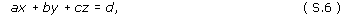where a, b, c and d are real, and a, b and c are not all zero.

Substituting the coordinates of each of the points in turn into equation (S.6), we obtain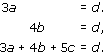From the first two equations we obtain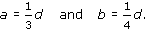Substituting these expressions into the third equation, we obtain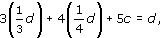so

d + d + 5c = d,

hence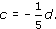Substituting these expressions for a, b and c into equation (S.6), we see that the equation of the plane can be written as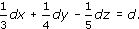Dividing by d, we obtain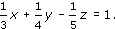It follows that one possible normal vector is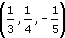.

M208_1